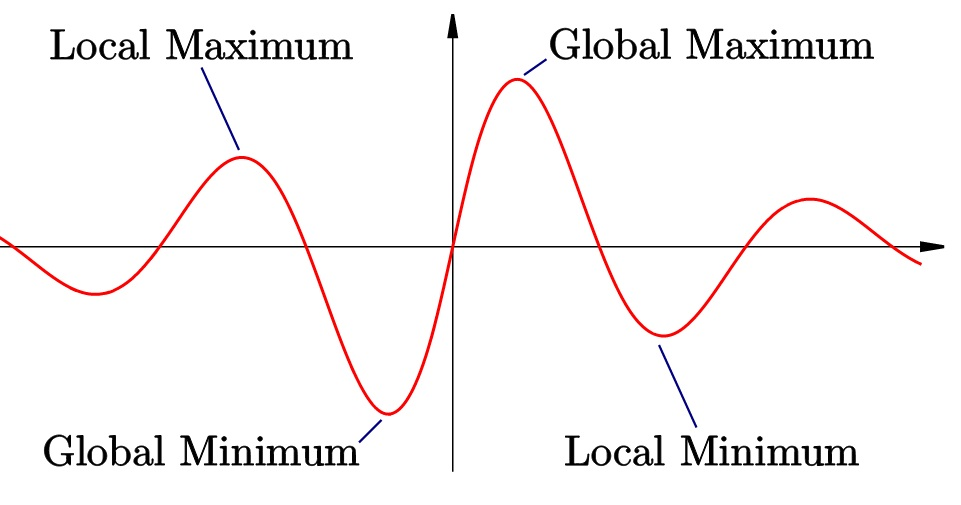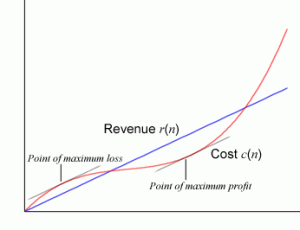# How to Find Maximum Profit (Profit Maximization)

Calculus Problem Solving > > How to find maximum profit

How to Find Maximum Profit: Contents:To maximize a function means to find its maximum value in a given range of values.

## How to Find Maximum Profit: Overview of Maximization

There are two ways to find maximum profit: with a graph, or with calculus.

Graphically, you’re looking for a global maximum.

With calculus, you can find the derivative of the function to find points where the gradient (slope) is zero, but these could be either maxima or minima.

• If the slope is increasing at the turning point, it is a minimum.
• If the slope is decreasing at the turning point, then you have found a maximum of the function.

## Profit Maximization given Profit and Cost Functions

Watch the video for a step by step example:

Profit maximization is one of the topics that are likely to be tested in the short-answer section of the AP Calculus exam. It is equal to a business’s revenue minus the costs incurred in producing that revenue. Profit maximization is important because businesses are run in order to earn the highest profits possible. Calculus can be used to calculate the profit-maximizing number of units produced.

## Find the maximum profit in calculus: Business ExampleExample question: Find the profit equation of a business with a revenue function of 2000x – 10x2 and a cost function of 2000 + 500x.

Step 1: Set profit to equal revenue minus cost. For example, the revenue equation 2000x – 10x2 and the cost equation 2000 + 500x can be combined as profit = 2000x – 10x2 – (2000 + 500x) or profit = -10x2 + 1500x – 2000.

Step 2: Find the derivative of the profit equation (here’s a list of common derivatives). For example, the profit equation -10x2 + 1500x – 2000 becomes -20x + 1500.

Step 3: Set the equation equal to zero:
-20x + 1500 = 0

Step 4: Use algebra to find how many units are produced from the equation you wrote in Step 3.
20x = 1500
x = 75.

Step 5: Calculate the maximum profit using the number of units produced calculated in the previous step. In this example, inserting x = 75 into the profit equation -10x2 + 1500x – 2000 produces -10(75)2 + 1500(75) – 2000 or 54,250 in profit.
That’s how to find maximum profit in calculus!

Tip:
For answering this type of question on the AP calculus exam, be sure to record this figure using the unit of measurement presented in the short-answer problem. For profit maximization short-answer problems on the AP Calculus exam, this unit of measurement is almost certainly US dollars or \$. Some equations might present more than one possible answer. Some of these answers can be picked out and discarded using common sense but most often cannot be treated the same. In these cases, insert all possible answers into the profit equation to calculate their profits and then select the answer that produces the highest profit as the profit maximizing number of units produced.

## How to Find Maximum Profit: Example with a Function and Algebra

Example problem: Find the local maximum value of y = 4x3 + 2x2 + 1.

Step 1: Differentiate the function, using the power rule. Constant terms disappear under differentiation.
d/dx (4x3 + 2x2 + 1) = 12x2 + 4x
The result, 12x2 + 4x, is the gradient of the function. This has two zeros, which can be found through factoring.
12x2 + 4x = 4x(3x+1), which equals zero when x = 0 or x = -1/3

Step 2: Check each turning point (at x = 0 and x = -1/3)to find out whether it is a maximum or a minimum. To do this, differentiate a second time and substitute in the x value of each turning point.
d/dx (12x2 + 4x) = 24x + 4
At x = 0, 24x + 4 = 4, which is greater than zero. This is a minimum.
At x = -1/3, 24x + 4 = -4, which is less than zero. This is a maximum.

Step 3: Find the corresponding y-coordinates for the x-value (maximum) you found in Step 2 by substituting back into the original function.
At x = -1/3, y = 4x3 + 2x2 + 1 = -4/27 + 2/9 + 1 = 29/27
Therefore the function has a maximum value at (-1/3, 29/27).

Tip: You can check your answer by sketching the graph and looking for the highest and lowest points.

## Finding Minimum Profit

One of the many practical applications of calculus comes in the form of identifying the maximum or minimum values of a function. Finding that minimum value is how to find minimum profit.

If you’ve spent any time at all in the world of mathematics, then you’ve probably seen your fair share of graphs with attached functions. Many graphs have certain points that we can identify as ‘maxima‘ and ‘minima‘, which are the highest or lowest points on a graph. It’s quite common to have a problem involving a function without an attached graph, so it can be useful to know the method behind getting these values.

## How to Find Minimum Profit with Calculus: Steps

Example Problem: Identify the minimum profits for company x, whose profit function is:
f(t) = 100t2 – 50t + 9,
where ‘f(t)’ is the money gained and ‘t’ is time.

Step 1: Differentiate your function. While the function itself represents the total money gained, the differentiated function gives you the rate at which money is acquired. This will be useful in the next step.
Here, I’m using the power rule:
f(t) = 100t2 – 50t + 9 is differentiated to become f ‘(t) = 200t – 50.

Step 2: Set the equation equal to zero and solve for t. 0 = 200t – 50
→ 50 = 200t,
Solving for t, you get t = 1/4.
This value means that there is either a maxima or a minima at t = 1/4.

Step 3: Test the surrounding values of t (in your original equation) to decide whether your value is a maxima or a minima.

1. Plug in your value for ‘t’ in the original equation.
For t = 1/4:
f(t) = 100(1/4)2 – 50(1/4) + 9 = 2.75.
2. Pick two very close points to the location of our extrema (t = 1/4). It is important to pick one value greater than and one less than your extrema. Typically, it is wise to pick quick and easy values for this part of the procedure. For instance, 0 and 1 are great choices, not only because they are very close, but also because they will allow you to do the computation in your head.
At t = 0, the equation becomes f(t) = 100(0)2 – 50(0) + 9 = 9.
At t = 1, the equation becomes 100(1)2 – 50(1) + 9 = 59.

Step 4: Compare the results. If you were to plot your three data points, it would look something like this:
(0, 9), (1/4, 2.75), (2,59).
You should be able to quickly draw a rough sketch of what this looks like – what you’ll find is that there is a minimum at 1/4. This fact is supported by the fact that the data points immediately to the left and the right of this value are both higher. If they were lower, the point would be a maxima, and if one were higher and the other lower, it would just be a point where the slope of the function is zero.

That’s how to find minimum profit!

Warning: Finding the minima of a function is fairly straightforward – but beware, in more complex equations, it can be quite difficult to obtain all of the values for ‘t’ where the function equals zero. In the event that there are multiple values for ‘t’, simple trial and error will lead the way to your minima or maxima.
Note:Step 2 at first seems a little strange, but remember that the derivative of a function represents the rate of the increase or decrease of the original function. If the function representing this rate is equal to zero, that means the actual function is not increasing or decreasing at that specific point. It is likely that at the point where the slope is zero, there will either be maxima or minima to identify.

## How to Find Maximum Profit: References:

http://earthmath.kennesaw.edu/main_site/review_topics/economics.htm Retrieved July 12, 2015.

CITE THIS AS:
Stephanie Glen. "How to Find Maximum Profit (Profit Maximization)" From StatisticsHowTo.com: Elementary Statistics for the rest of us! https://www.statisticshowto.com/calculus-problem-solving/find-maximum-profit/